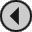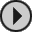## 3D CONSTRUCTION KIT

### HOW TO USE VARIABLES

The format for using a VARIABLE can be handled in the same way through various types of conditions. We could for example, arrange for a variable to be increased to hold a higher value when an object is shot, as follows:
```   IFSHOT 3
THEN
```
Thus adding 25 to the VARIABLE number 21. In a similar way a value can be deducted from a VARIABLE using the following example:
```   IFSHOT 3
THEN
SUBV 15 21
```
To set a VARIABLE to hold a specified number we could use the following GENERAL condition commands:
```   SETV 30 21
```
This same process can be incorporated into slightly more complicated conditions where we want to check the value of the variable and then if TRUE to set the variable to hold another value as follows:
```   CMPV 0 21
IFLT
THEN
SETV 30 21
ENDIF
```
Thus if Variable 21 holds a value greater than 0, Variable 21 will be set to hold the value 30.

The use of variables enables you to create a wide range of conditions, from the very simple to the complicated. The system has 112 (0-111) variables available for use by the COMMAND LANGUAGE. These variables are 8 bit storage areas (that is they can hold numbers in the range 0-255, which can be used to store and manipulate various numerical values within the environment e.g. player score, fuel supply or a timer. 16 of the available Variables (112 to 127) are used by the FREESCAPE II system. The contents of these variables are updated each frame by the system, and any changes to the variables are so noted by the system i.e. if a variable command were to change the value stored in variable 112 (the Viewpoint X position (low)) the next displayed frame would move the player to the new specified X position. A list of the contents of the system variables follows:
112 = Viewpoint X position Low
113 = Viewpoint X position High
114 = Viewpoint Y position Low
115 = Viewpoint Y position High
116 = Viewpoint Z position Low
117 = Viewpoint Z position High
118 = Viewpoint X Rotations (0-71 increments of 5 degrees)
119 = Viewpoint Y Rotations (0-71 increments of 5 degrees)
120 = Viewpoint Z Rotations (0-71 increments of 5 degrees)
121 = Current key presses (ASCII)
122 = Interrupt counter Low
123 = Interrupt counter High
124 = Current Area/Data set
125 = Ammo counter
126 = Font pointer starting at character 32 Low
127 = Font pointer starting at character 32 High

Note 1:
Variable 125 (Ammo counter) - If set to 0 the player cannot fire. If set to 255 then player has infinite ammo else decremented per shot.

Note 2:
Variables 126 and 127 (Font pointers) are for advanced users only.